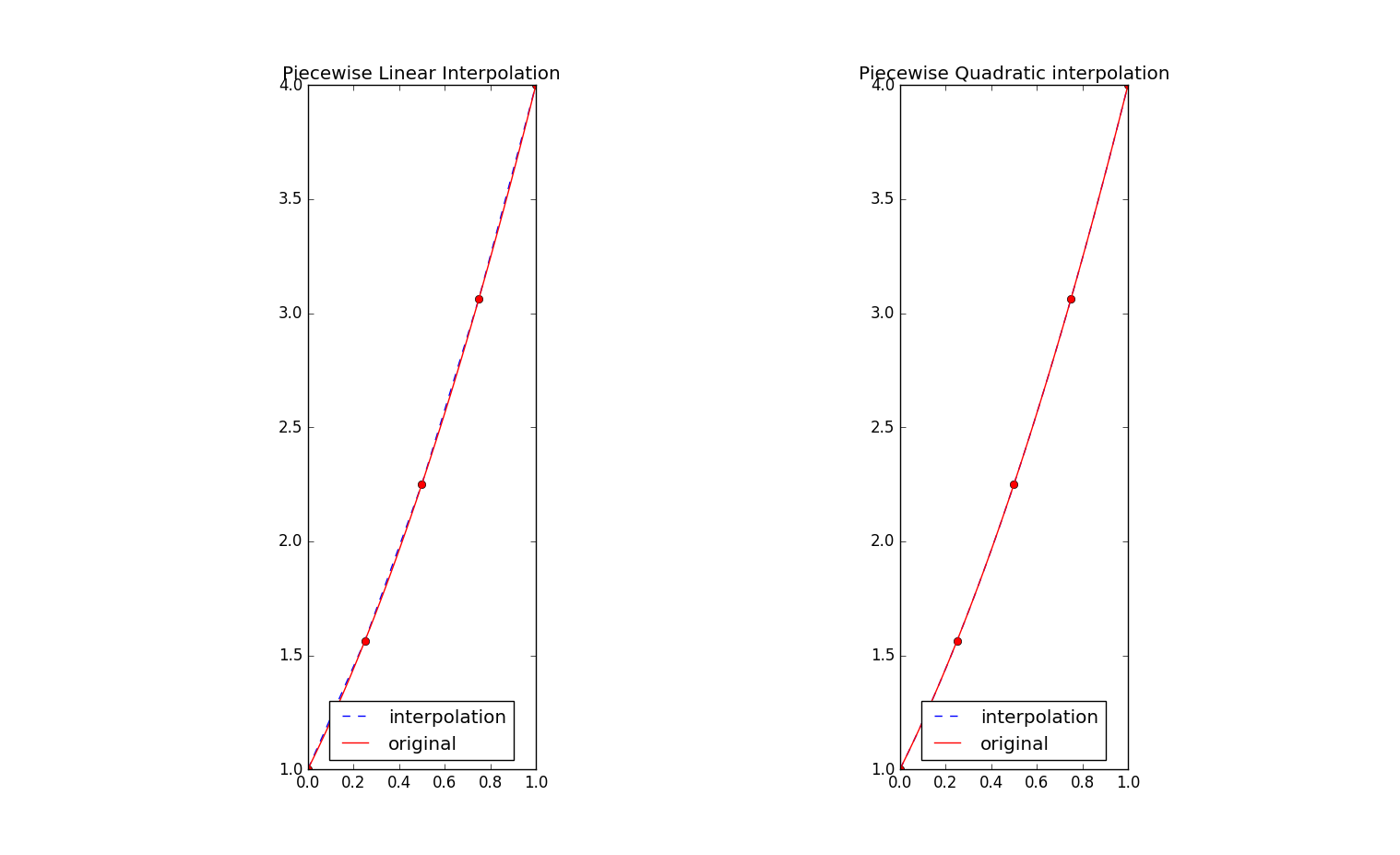# 14 SciPy分段多项式插值

## 14.2 SciPy分段多项式插值示例

• xi列表在$[0,1]$上取5个点，通过numpy的linspace函数构造出来。
• yi需要给出各个xi的0次和1次导数值。
• 由于打算做二次多项式插值，那么各段插值多项式都是二次的，故此时的order可以设置为一个纯的数2,如果打算在各个区段用不同的次方的多项式来插值，则可以用列表给出各个段上的多项式的最大值。

yi在xi上的0次导数实际就是f(x)本身，而1次导数为f'(x) = 2x + 2, 2次导数 f''(x) = 2

#coding:utf-8
from scipy.interpolate import PPoly,interp1d,PiecewisePolynomial
import numpy as np, matplotlib.pyplot as plt

nodes = np.linspace(0, 1, 5)
x = np.linspace(0, 1, 100)
def f(t):
return t**2 + 2*t + 1
print "nodes", nodes
print "f_nodes", f(nodes)
#分段一次插值
interpolant = interp1d(nodes, f(nodes), kind='linear')
plt.figure(figsize=(12,6))
plt.subplot(121, aspect='equal')
plt.plot(x, interpolant(x), 'b--', label="interpolation")
plt.plot(nodes, f(nodes), 'ro')
plt.plot(x, f(x), 'r-', label="original")
plt.legend(loc=8)
plt.title("Piecewise Linear Interpolation")

#分段二次插值
yi = np.zeros((len(nodes), 2))
yi[:,0] = f(nodes)#0次导数
yi[:,1] = 2 * nodes + 2#1次导数
print yi
#每个区段都是2次多项式插值
interpolant = PiecewisePolynomial(nodes, yi, orders=2)
print "->",interpolant(0.25)
#每个区段用不同的次的多项式插值
#interpolant = PiecewisePolynomial(nodes, yi, orders=[2,1,2,1])
print help(interpolant)
#给出
print "->",interpolant.derivatives(0.6)
plt.subplot(122, aspect='equal')
plt.plot(x, interpolant(x), 'b--', label="interpolation")
plt.plot(nodes, f(nodes), 'ro')
plt.plot(x, f(x), 'r-', label="original")
plt.legend(loc=8)